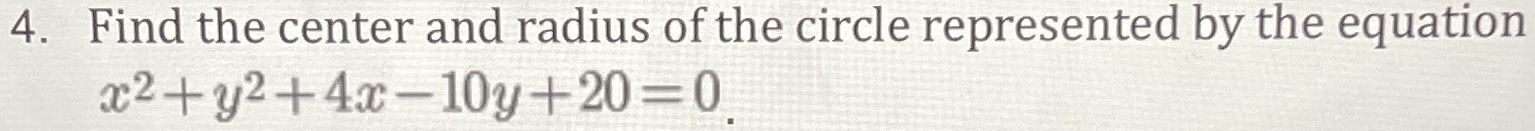### ¿Todavía tienes preguntas de matemáticas?

Pregunte a nuestros tutores expertos
Algebra
Pregunta4. Find the center and radius of the circle represented by the equation

$$x ^ { 2 } + y ^ { 2 } + 4 x - 10 y + 20 = 0$$

$$x ^ { 2 } + y ^ { 2 } + 4 x - 10 y + 20 = 0 : \quad$$ Circle with center at $$( - 2,5 )$$ and radius $$r = 3$$